#Quality Digest

Featured Product
This Week in Quality Digest Live
Six Sigma Features
Scott A. Hindle
Part 4 of our series on SPC in the digital era
Donald J. Wheeler
What are the symptoms?
Douglas C. Fair
Part 3 of our series on SPC in a digital era
Scott A. Hindle
Part 2 of our series on SPC in a digital era
Donald J. Wheeler
Part 2: By trying to do better, we can make things worse
Six Sigma News
How to use Minitab statistical functions to improve business processes
Sept. 28–29, 2022, at the MassMutual Center in Springfield, MA
Elsmar Cove is a leading forum for quality and standards compliance
Is the future of quality management actually business management?
Too often process enhancements occur in silos where there is little positive impact on the big picture
Collect measurements, visual defect information, simple Go/No-Go situations from any online device
Good quality is adding an average of 11 percent to organizations’ revenue growth
Floor symbols and decals create a SMART floor environment, adding visual organization to any environment
A guide for practitioners and managersBio

Six Sigma

## Working with Rare Events

### What happens when the average count gets very small?

Published: Friday, October 28, 2011 - 08:23

From the perspective of data analysis, rare events are problematic. Until we have an event, there is nothing to count, and as a result many of our time periods will end up with zero counts. Since zero counts contain no real information, we need to consider alternatives to counting the rare events. This article will consider simple and complex ways of working with rare events.

Our first example will involve spills at a chemical plant. While spills are not desirable, and while everything possible is done to prevent them, they do occasionally happen. Over the past few years one plant has averaged one spill every eight months. Of course, if the plant averages one spill every eight months, then those months with a spill will be 700 percent above average! (When dealing with small counts a one unit change can result in a huge percentage difference.) Assuming that these counts are reasonably modeled by a Poisson distribution we could put these counts on a c-chart. The central line for this c-chart would be the average count. During the first four years there were a total of six spills. Six spills in 48 months gives an average of 0.125 spills per month.

For a c-chart the upper limit is found by multiplying the square root of the average count by 3.0 and adding the result to the central line. This gives the upper limit of 1.186 shown on the c-chart in figure 1.Figure 1: The number of spills per month on a c-chart

In spite of the fact that a single spill is 700 percent above the average, the c-chart does not show any points outside the limits. Here it would take two spills in a single month to make this chart signal a change. (Only 1500 percent above average!) So, while the use of a c-chart might be justified with these counts, it is of little practical use because it is so insensitive.

What about using an XmR chart with these counts as I suggested last month? Using the first four years as our baseline the moving range chart would have an upper limit of 0.83 and the X chart would have an upper limit of 0.80. This makes every month with a spill into a signal of a change in the system! Clearly this is not a reasonable interpretation of these data. The problem is that this XmR chart suffers from the problem of chunky data. (Chunky data can occur with any type of data. Count data tend to be chunky whenever the average count falls below 1.00. Chunky data will artificially tighten the limits of a process behavior chart and will result in an excess number of false alarms. More about the problem of chunky data next month.)

Thus, with counts of rare events the specialty charts become insensitive and the XmR chart breaks down. This is not a problem with the charts, but rather a problem with the data themselves. Counts of rare events are inherently insensitive and weak. No matter how these counts are analyzed, there is nothing to discover by placing the counts on a chart of any type. Yet there are other ways to characterize rare events. Instead of counting the number of spills each month (counting events), you could instead measure the number of days between the spills (measure the area of opportunity between the rare events). For these data the time intervals between the spills are computed as follows.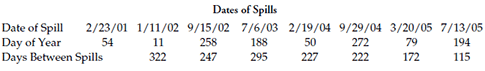Figure 2: Determining the time between spills

One spill in 322 days converts into a spill rate of 0.0031 spills per day. Multiplying this daily spill rate by 365 gives us a yearly spill rate of 1.13 spills per year. Thus, the interval between the first spill and the second spill is equivalent to having spills at the rate of 1.13 spills per year. In the same way the interval of 247 days between the second and third spills is converted into a spill rate of 1.48 spills per year. Continuing in this manner, every time we have an event we obtain an instantaneous spill rate.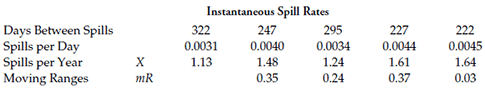Figure 3: Instantaneous spill rates and moving ranges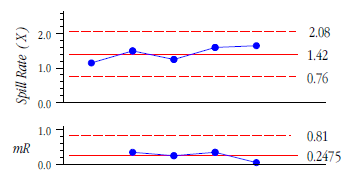Figure 4: XmR chart for spill rates

The average spill rate during the first four years is 1.42 spills per year. The average moving range is 0.2475. Multiplying this latter value by 2.66 and adding and subtracting the result to and from the 1.42 we get the X chart limits shown in Figure 4. The upper range limit is found by multiplying the average moving range by 3.27. While the use of five values to create an XmR chart is minimal, it took four years to get these five values!

If a future point falls above the upper limit it will mean that the spill rate is increasing. A future point below the lower limit will mean that the spill rate is decreasing. Points within the limits will be interpreted as meaning that there has been no change in the overall spill rate. The two spills in the current year had intervals of 172 days and 115 days respectively. These intervals convert into spill rates of 2.12 spills per year and 3.17 spills per year. When these values are added to the XmR chart the result is figure 5.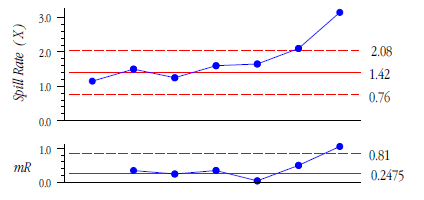Figure 5: Complete XmR chart for spill rates

While the first spill in the current year is outside the limit, it is barely outside. Given the softness of limits based on five values, we might be slow to interpret the sixth point as a clear signal of a change. However, the seventh point is far enough outside the limits to be safely interpreted as a definite signal—there has been an increase in the spill rate during the current year. If we return to figure 1 we can see that the spills are getting closer together, but we cannot detect this change until we shift from counting the rare events to measuring the area of opportunity between events.

Notice that while both figure 1 and figure 5 are looking at spill rates, there has been a change in the variable between figure 1 and figure 5. In figure 1 the variable was the number of spills per month. Here the numerator was allowed to vary (the umber of spills) while the denominator was held constant (one month). In figure 5 we have instantaneous spill rates where the numerator is held constant (one spill) and the denominator is allowed to vary (days between spills).Figure 6: XmR chart for days between spills

Instead of using the instantaneous spill rates, figure 6 uses the number of days between spills to create an XmR chart. While this is feasible, this chart suffers from being the chart of an inverse measure. As the spills become more frequent the points in figure 6 move downward. This simple inversion creates a cognitive dissonance for those who have to interpret this chart. While this is not an insurmountable obstacle, it is still a hurdle that is unnecessary here. The instantaneous spill rates of figure 5 are easier to use and easier to interpret than the number of days between spills in figure 6.

In addition to being an inverse measure, the time between events results in a chart that is less sensitive than the chart for the instantaneous spill rates. Figure 5 will detect an increased spill rate whenever that rate exceeds 2.08 spills per year. The lower limit of figure 6 corresponds to a spill rate of 2.76 spills per year. Given that these are techniques for rare events and that we want to detect any increase in the spill rate in as timely a manner as possible, this lower sensitivity of figure 6 is undesirable.

While the chart for the instantaneous rates will generally be the preferred chart, there is one situation where the chart for the times between events is useful. This is when the lower limit of figure 5 goes below zero. When this happens the instantaneous rate chart will no longer show improvements. If you are involved in taking action to reduce the rate of the rare events, so that detecting improvements is important, then you may need to resort to charting both the instantaneous rates and the time between events. The chart for instantaneous rates will allow you to detect increases in the rate of rare events, while the chart for the time between events will allow you to detect decreases in the rate as points above the upper limit. This will be illustrated by the next example.

Figure 7 contains the number of consecutive cases between post-operative sternal wound infections in one cardiac care unit. These data represent 3106 patients treated at this one facility. With a total of 75 sternal wound infections this unit has an overall infection rate of 2.4 percent. While this summary statistic describes the past, the question of interest is whether we can use it as a prediction of what to expect in the future. To answer this question we will need to look at the way the data behave over time. Figure 7 should be read in columns.Figure 7: Counts of cases between infections for a coronary care unit

The X chart for these counts is shown in figure 8. Here the median count is 26 (which corresponds to an infection rate of 3.8%) and the median moving range is also equal to 26. Multiplying the median moving range by 3.145, and adding the result to the median value of 26 results in an upper limit of 107.8. The points above this upper limit represent eight periods where the infection rate was detectably lower than the rate of 3.8 percent associated with the median count of 26. So while this chart detects eight periods of improved operation, these data will not allow us to detect any increase in the infection rate of this coronary care unit.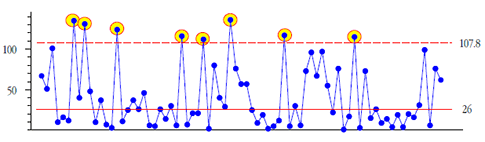Figure 8: X chart for the number of cases between infections

In order to detect periods of increased infection rates we will need to invert the counts of figure 7 to obtain instantaneous infection rates. The X chart for these rates is shown in figure 9. The median rate is 0.040, and the median moving range is 0.056. Here we find seven periods where the infection rate was detectably higher than four percent. (The eight periods with detectably lower infection rates from figure 8 are also shown as circled points along the bottom of figure 9.)

All in all, these charts tell a story of a hospital that is not yet in control of all of the potential sources of infection in its coronary care unit. While their historical average infection rate has been 2.4 percent, this 2.4 percent is not a characteristic of a predictable process, but merely the average over periods with higher and lower infection rates.Figure 9: X chart for the instantaneous infection rates

Thus, between these two charts we can identify periods of higher than average infection rates and periods with lower than average infection rates. Unfortunately, we can do so only after the fact. Because these charts do not add a point until after an event has occurred, they will always remain essentially report card charts.

The vertical scale of figure 9 is nonlinear on the upper end simply because there are only certain values that occur when we invert counts smaller than 10. This is a natural consequence of having areas of opportunity that are counts.

In figure 8 and figure 9, I used the median moving range to compute the limits because the average moving ranges were inflated by the extreme values and did not capture the routine variation within the data. I also used the medians as the central line in both X charts simply because the averages fell at the 64th and 73rd percentiles respectively, making them poor measures of location.

### Specialty charts for times between events

In the 1990s, specialty charts for the times between events were created. These charts are commonly known as g-charts and t-charts. As with the p-chart, np-chart, c-chart, and u-chart discussed last month, these charts use a probability model to construct theoretical limits for the times between events. However, unlike the traditional specialty charts, the g-chart and t-chart have an implicit assumption of global homogeneity built into the computations. This assumption of homogeneity is equivalent to the erroneous computation of limits for a regular process behavior chart using the global standard deviation statistic. (See my columns for January and February of 2010 for more on this mistake.)

The g-chart is based on a geometric distribution, and is applied to counts like those in figure 7, the number of items observed between items having some attribute. The use of this probability model once more allows the computation of a theoretical three-sigma distance directly from the average value. In the case of figure 7 the average value is 41.4. The theoretical three-sigma distance is found by squaring the average value, subtracting the average from this squared value, taking the square root, and multiplying by 3 to get 122.7. When this quantity is added to the average value we find an upper limit of 164. The resulting g-chart is shown in figure 10.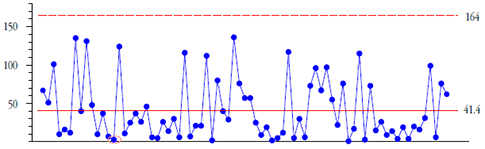Figure 10: G-chart for number of cases between infections

While the nature of these data preclude this chart from detecting when things get worse, the assumption of global homogeneity makes it difficult for this chart to detect when things get better. So while any chart for the time between events will usually be a report-card chart, this g-chart suffers from an inability to tell you when things are changing (compare figure 10 with figure 8).

Before the counts in figure 10 can be considered to be geometric counts, the series of cases will need to be a series of Bernoulli events where the probability of an infection remains the same for all of the cases in the baseline period. In short, the computations for the limit of figure 10 assume the data set to be homogeneous. (A series of n Bernoulli events will result in a binomial count only when the probability of the counted outcomes p remains constant across the n events. A series of values for the numbers of Bernoulli events between counted outcomes will be a geometric variable only when p remains the same for each of the intervals. Thus, in order to use the geometric probability model to obtain appropriate limits for the data in figure 10, the probability of an infection must remain the same throughout the baseline period. This assumption is inconsistent with these data and unrealistic for the context of these data.) Any use of a g-chart imposes an assumption of homogeneity upon the data prior to looking for evidence of a lack of homogeneity. As such it is an example of the triumph of computations over common sense. It simply does not provide any opportunity to learn from the data.

Now before you write to tell me how your g-chart had points above the upper limit, you need to know that the geometric distributions will have an absolute minimum of 1.8 percent false alarms with three-sigma limits, and that some of these false alarm points will be comfortably beyond the upper limit. (It is interesting to note that those who want to transform data in order to avoid having a false alarm rate of one or two percent on an XmR chart do not make the same objection to the g-chart. Perhaps they are simply unaware of the properties of the techniques they are using.)

### The t-chart

While the g-chart was intended for those cases where the time between events is a count, the t-chart was intended for those cases where the time between events is a measurement. For an example return to the first example where the spills occurred in time and the time between events was measured in days. The t-chart would use the times between spills (as shown in figure 2) as the raw data.

Now there are two different versions of the t-chart. One version uses a probability model to compute theoretical limits and the other version transforms the data and places the transformed data on an XmR chart. In both versions the initial assumption is that the rare events are characterized by a Poisson probability model. (This means that the likelihood of an event is proportional to the size of the area of opportunity and that the rate of occurrence for the rare events is constant throughout the area of opportunity.) Next, given that the counts are Poisson, the times between events will be modeled by an exponential distribution. The mean value for these exponential variables will depend upon the rate of occurrence of the Poisson events. Both versions of the t-chart assume that the times between events come from one and the same exponential distribution. (In order for all of these exponential variables to have the same mean value the Poisson rate must remain constant across all the occurrences.) The first version uses this assumption of global homogeneity to create theoretical limits for the data.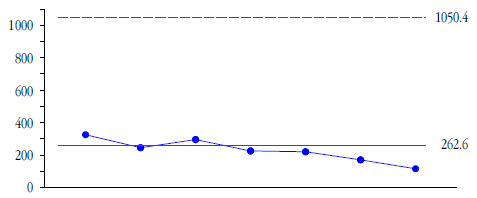Figure 11: Simple t-chart for times between spills

The exponential distribution starts at zero and has a standard deviation that is equal to the mean. This means that there will be no lower limit, and that the upper three-sigma limit will be equal to four times the mean value. Thus, in constructing this version of the t-chart, the central line will be equal to the average time between events, and the theoretical upper limit will be equal to four times the average value. Using the first five times between events of figure 2 as our baseline we get figure 11. Compare this with the graph in figure 6. The absurdity of figure 11 is sufficient to warn the experienced analyst that these data are not exponentially distributed and that the theoretical approach based on the assumption of an exponential distribution is incorrect.

The second version of the t-chart still assumes that all of the times between events in the baseline period come from a single exponential distribution, but this version transforms the data prior to placing them on a chart. Since exponential random variables can be converted into Weibull random variables by a power transformation, and since a Weibull distribution with parameter 3.6 has zero skewness, the times between events are all raised to the 1/3.6 = 0.27778 power in order to remove the assumed skewness from the data. Then these transformed values are placed on an XmR chart.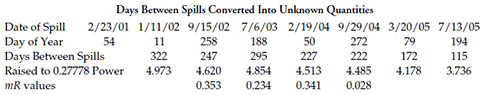Figure 12: How your data can become lost in probability space

As before we use the first five intervals as our baseline and place the transformed intervals on an X chart. The average is 4.689 and the average moving range is 0.239. This gives the chart and limits shown in figure 13. The limits here are substantially different from figure 11 because these limits are empirical limits computed from the transformed data, rather than being theoretical limits computed for an assumed probability model.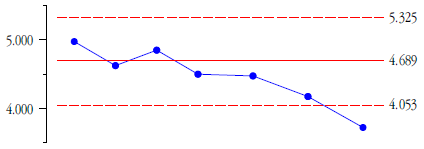Figure 13: Complex t-chart for transformed times between spills

As in figure 6 the last point in figure 13 falls below the lower limit signifying an increase in the spill rate. However, unlike either figure 5 or figure 6, the chart in figure 13 assumes that the rate of occurrence for the spills is constant over the whole baseline period. While this may be reasonable for these data, it will not always be so. This assumption of a constant rate is particularly troublesome when we are using these report chart charts to evaluate improvement efforts that will hopefully change the rate of occurrence for the rare events. (At the minimum, this assumption will argue against the use of long baselines for computing limits.)

For a final example I shall use a data set coming from a document in my possession. It is the time in days between the occurrences of an unspecified (unfavorable) event at a hospital. The times are shown in figure 14. In the document the data were all raised to the 0.27778 power, placed on an XmR chart, and the limits were computed using all of the data. (This corresponds to a baseline of 4.5 years.) The average moving range for the transformed values is 0.751, which gives the limits shown in figure 15.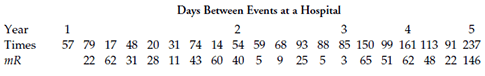Figure 14: Events per year and time between events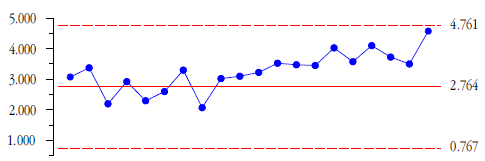Figure 15: Complex t-chart for transformed days between events

The only glimmer of a signal is the long run above the central line in figure 15. Unfortunately, when we transform the data in a nonlinear manner we effectively shift the central line relative to the rest of the data (compare the central lines with the running records in figures 15 and 16). This makes the interpretation of long runs tricky and undermines the use of the traditional run tests. While this run of 12 points is likely to be some sort of a signal, this interpretation is subjective and owes more to the following analysis than to the chart in figure 15.Figure 16: XmR chart for the days between events

If we simply place the days between events on an XmR chart we get the chart shown in figure 16. For this 4.5 year period the average is 81.9 and the average moving range is 38.8. The point above the limit and the run leading up to that point suggest that there was a change in the rate of these events sometime during Year Two. Moreover, the point above the upper range limit suggests that Year Five is going to have a detectably lower rate for this event than what was seen in Years Two, Three, and Four. However, waiting until you have over four years worth of data before computing limits is simply not realistic for these data. If we assume that an effort to reduce the rate of this event was started at the beginning of Year Two, we might have used Year One as our baseline. This would produce the chart and limits shown in figure 17.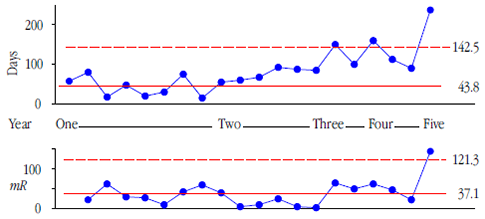Figure 17: XmR chart for the days between events with year one as baseline

Here we detect a lowered rate for this event in Year Three. Interpreting the run in which this point above the limit occurs we see that this improvement might have begun as early as the start of Year Two. And Year Five still looks like it will be better than the previous years. Contrast the clarity and interpretability of the signals in figures 16 and 17 with the lack of any clear signal in figure 15.

### Summary

Whenever the average count per time period drops below 1.00 you are working with rare events. When this happens the p-chart, np-chart, c-chart, and u-chart will all become very insensitive. At the same time the problem of chunky data will prevent you from using the XmR chart with the counts of items or the counts of events. When this happens you should shift from counting the events per time period and instead measure the area of opportunity between the rare events. Here you cease to get a value every time period, and instead get a value every time you have an event. (This shift in how you collect the data argues against using this approach except in the case of rare events.)

When working with the times between events you may wish to compute instantaneous rates for each event and place these on an XmR chart as illustrated in figures 4 and 5, or you may work directly with the times between events as shown in figure 6. When these charts become one-sided, you may need to work with both charts in order to detect both improvements and deterioration, as shown in figures 8 and 9.

While specialty charts have been created for times between events, they suffer the logical flaw of making a strong assumption that the data are homogeneous prior to examining those data for homogeneity. As a result the g-chart in figure 10 failed to show any of the signals found in figures 8 and 9, and the simple t-chart in figure 11 failed to detect the signal found in figure 6.

The complex t-chart does a better job than the simple t-chart simply because it uses empirical limits rather than theoretical limits. For this reason figure 13 found the same signal shown in figure 6. However, the complex t-chart still assumes that all of the times between events are modeled by one and the same exponential distribution, and it applies the same nonlinear transformation to all of the values prior to placing them on a chart. As is generally the case, this nonlinear transformation will tend to hide the signals within the data. Thus, figure 15 failed to show the signals found in figures 16 and 17.

The g-chart and the t-chart seek to provide exact solutions to specific problems.

Unfortunately, these specific problems do not match the realities of the data we actually encounter. The XmR chart simply takes the data as they exist and examines them to see if they show evidence of a change in the underlying process. This empirical approach may use approximate limits, but it is a robust approach that works with all types of data. (See my column for November of 2010 for more on this topic.) And as the famous statistician John Tukey once said: “It is better to have an approximate answer to the right question than an exact answer to the wrong question.”

### Discuss### Donald J. Wheeler

Dr. Wheeler is a fellow of both the American Statistical Association and the American Society for Quality who has taught more than 1,000 seminars in 17 countries on six continents. He welcomes your questions; you can contact him at djwheeler@spcpress.com.

The question addressed by any process behavior chart is more basic than "What is the shape of the histogram?" or "What is the probability model?"  It has to do with whether we can meaningfully use any probability model with our data.  In starting with distributional questions you beg the question of homogeneity which the chart was created to address.  This mistake was made by E. S. Pearson in 1935, and has been repeated many times since.  You need to reread Shewhart's two books more carefully.

### Distributions

Don - thank you for your reply to my comments.  This seems to be a chicken and egg situation.  If you assume data to be homogenous, then you may only detect a future issue that has deviated from the current homogenous state.  However, if the data were not homogenous to begin with, then this assumption may be masking signals.  So the best decision would seem to be to use some other knowledge to consider whether your assumption is reasonable or not.

In this case I would use two pieces of knowledge.  The first is that a Poisson process is known to have "opportunities between occurences" to be geometrically distributed.  In this case it may be reasonable to believe this is a Poisson process but we probably want other evidence as well.  Which would bring me to the second piece of knowledge which is the graph itself...removing centerline and control limits, the data simply look random.  As a practitioner, I would have serious doubts about the points labelled as out-of-control actually having special causes and would not waste time pursuing special causes for 20% of my points.

I have certainly read Shewhart's books and articles and understand he did not want control charts to be interpreted as fitted specific probabilities associated with the normal (or any other) distribution.  However, among all of the "example" datasets he uses, there is never a sample that comes out as skewed as data from time between events typically does.  They are all roughly "moundish".  I don't think considering data to have potentially come from a highly skewed distribution is rejecting Shewhart's ideas so much as evaluating an area in which Shewhart did not publish work.

In determing the best way to plot data from what we have good reason to believe is a highly-skewed distribution, the exponential or geometric distributions may not be perfect but they provide more reasonable limits than Shewhart's methods and only require one chart.  I find this to be much more useful and to provide more balanced and reasonable false alarm rates.

Also, I'm interested in investigating the methods you described for G- and T-Charts as they are not the ones I am familiar with.  Is there a reference I can consult for more information?  Your help would be greatly appreciated!

### Great article

I actually met Don Wheeler at an ASQ annual conference in 1996.  I went to his booth asking the question of what to do about rare events.  His answer was "Buy this book" (Understanding Variation The Key to Managing Chaos) and even got his autograph (though I am sad to report someone at some point borrowed the book, and I don't have it on hand).

I've used the rate between events chart described in the beginning of the article with good success.  It does get a bit hard to explain to management, as the average of the rates ends up being skewed higher than might be expected by the layman.  I am convinced this "skewing" is good for the analysis.

The charts do tend to (in my empirical experience) be a little more susceptible to false alarms than others, but in the safety arena I'd rather have a few more false alarms.

One suggestion I have, which removes the past looking "report card" feature is to plot a "ghost point" for today when the chart is updated.  That is - if an event were to happen 1 minute after I updated the chart, what would it look like?  That gives the context of how long have we gone since the last event.  Stretching things a little, I've even converted that to the exponential probability of what is the likelihood that we could have gone that long given the current average rate that is plotted.  That hasn't been as beneficial especially in layman conversations and may be questionable statistically, but might be worth considering.

Thanks,

### Rare Events and Safety Statistics

I have been using the methodology described by Dr. Wheeler to analyze Safety data (OSHA Recordable Injury Rates, in particular) for several years with good success in seeing the signals which are otherwise difficult to detect.

### Another Classic from the Master

Andrew Torchia Principal Quality Consultant www.qaexp.com

Once again Dr. Wheeler condenses a chapter's worth material into a single column that is highly educational yet easy to read.

### A classic?

Looking at the graph of infection rates, my instincts told me that the process was stable as nothing about it looked nonrandom.  So I did some fact-checking, and the data certainly appears to fit the distributional assumption of geometric (and distribution fit is important for G and T Charts).  So then I took Dr. Wheeler's control limits, and calculated the odds of randomd data from that geometric distribution falling outside of the control limits - the result: 18.3%.  18.3%!  That's your false alarm rate.  He then circles 20% of the points and claims there is a lack of control.

If you think this is "a classic" then have fun chasing false alarms all day.  Apparently no research at all was done on the properties of the method he is proposing and as a statistician, I am deeply concerned about anyone reading this and blindly believing this is a better method.  As this method additionally requires calculations on your data, I do not see how it is easier either.

Further, the methods described for how standard G and T Charts are constructed are not common methods, and chances are very good that your statistical package uses a much better method and does not require the use of two different charts to detect shifts up and down.

If you have this type of data, check to see if it meets the distribution assumption of geometric or exponential (just a histogram should be a good enough check).  If it does, use the standard G or T Chart that Minitab or other packages provide.  If it does not then it is most likely more symmetric, in which case an I-MR Chart would work fine although you should ensure you have a posisitive LCL.